The Egyptians wrote in hieroglyphs using symbols for both numbers and words

There were different hieroglyphs for 1, 10, 100, 1,000, 10,000, 100,000 and 1,000,000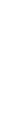=1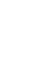= 10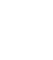= 100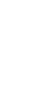= 1,000 Loading...Loading...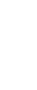= 10,000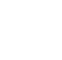= 100,000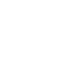= 1,000,000

Numbers were written with the highest number first

For example:

 1,111 =200,250 =1,122,132 =*Main image by Jeff Dahl